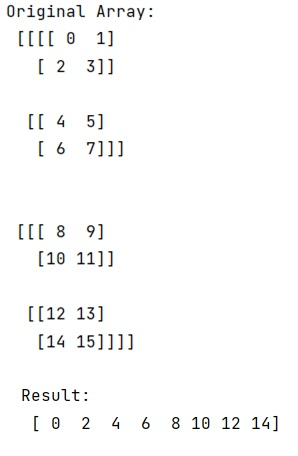# What does 'three dots' mean when indexing what looks like a number?

Learn about the meaning of 'three dots' when indexing what looks like a number?
Submitted by Pranit Sharma, on December 26, 2022

NumPy is an abbreviated form of Numerical Python. It is used for different types of scientific operations in python. Numpy is a vast library in python which is used for almost every kind of scientific or mathematical operation. It is itself an array which is a collection of various methods and functions for processing the arrays.

## Meaning of 'three dots' when indexing what looks like a number

In Python, the three dots indexing is often termed ellipses indexing. The ellipsis is used in NumPy to slice high-dimensional data structures. It is designed to mean at this point, inserting as many full slices (:) to extend the multi-dimensional slice to all dimensions.

There is another use for ellipsis, which has nothing to do with slices. It can be used in intra-thread communication with queues, as a mark that signals "Done".

Let us understand with the help of an example,

## Python code to demonstrate the 'three dots' mean when indexing what looks like a number

```# Import numpy
import numpy as np

# Creating a numpy array
arr = np.arange(16).reshape(2,2,2,2)

# Display original array
print("Original Array:\n",arr,"\n")

# Using the three dots indexing
res = arr[..., 0].flatten()

# Display result
print("Result:\n",res,"\n")
```

Output: